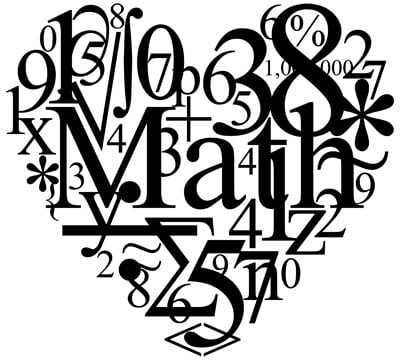Tuesday, October 19, 2021HomeLifestyle-65÷7, Remainder -2? Wrong. That And Other Basic Maths You Are Getting...

# -65÷7, Remainder -2? Wrong. That And Other Basic Maths You Are Getting Wrong

-

By Rusel D’cruze

1. The value of pi: 22/7 or 3.14159265….?Put straight, the actual value of pi is 3.141592653589793238462643383279502884197169399375105820974944…

THAT is what you get when you divide the circumference of a circle by its diameter. That’s pi.

The number 22/7 is just the nearest approximation.

Look at this:

22/7 = 3.142857142857142857….

That is clearly not exactly pi, but very close to pi. Also, in the actual value of pi, the numbers after the 3 do not follow any pattern known so far and do not end.

In the above number, the sequence 142857 repeats indefinitely. More accurate calculations place 355/113  at a higher level of accuracy than 22/7. However, 22/7  is commonly used so that you don’t groan if you’re not allowed a calculator.2. The Square root of 4. 2, -2 or both?

Throughout this section, I’ll refer to the square root of 4 as sqrt(4).

Most people to whom I ask this question say that sqrt(4) is simultaneously 2 and -2 as both values give you 4 when squared (multiplied with themselves). Hence, sqrt(4) is thought of as any number which yields 4 when squared.

But the symbol √ (square root) is not like + (plus) or – (minus), it’s a function (I’ll explain).

So, the rules say a function MUST NOT have more than one ‘output’ for a particular ‘input’ (i.e. 4).

(You don’t break rules or you’re screwed on test day). So, you can’t have two outputs, 2 and -2.

The question you might ask is why take sqrt(4) to be positive, why can’t it be -2 instead of 2?

The answer might sound unreasonable, but this is the convention accepted throughout Mathematics (yeah and you thought life was unfair).

Therefore, we can summarize the above discussion into this simple line,

Sqrt(x)  is not the number which when squared gives x, it is the POSITIVE number which when squared gives x.”3. Let’s see if you know your division!

Have a look at this division:Chill! Messing with you, it’s fine. 65 divided by 7, 2 as the remainder.

Also, look at the following division.

-65 – (-63) = -2The above division may appear just fine, but remember the good ol’ “Dividend is equal to divisor into quotient plus remainder. Well, a condition on that is that the remainder needs to be non-negative, i.e. 0 or more than it.

Note the small difference between the terms ‘positive’ and ‘non-negative’. Non-negative numbers include 0, positive numbers don’t. Since the remainder is negative, the above division is wrong.

Here’s what you would do normally. Multiply the divisor with a number less than the dividend, 63 is less than 65 and hence, it is suitable to multiply the divisor 7 with 9.

However, in the second division, -63 is more than -65 so you shouldn’t be multiplying 9 with 7. Instead multiply -10 with 7 to obtain -70 which is the nearest number less than -65 and the ensuing division would look like this:

-65 – (-70) = 5A non-negative remainder!

So, remember this little devil when you’re dividing negative numbers.

Alright! We’re done here. Thanks for reading; you are mathematically enlightened now just in case you weren’t aware of those little mistakes people make.

Spread that light I just gave you in case you meet someone who doesn’t know.

(Imma be theological! Hate the sin, not the sinner)

### Amid an outburst of a looming energy crisis, let’s meet an...

October 19: There are grim reports of a looming energy crisis in India. Every day there is a lot of speculation in the media...

### Lead Actress Of Punjabi Song “Pre Dreams” Priya Singh Khatri Is...•
• Or, Like us on Facebook

Subscribe to India’s fastest growing youth blog
to get smart and quirky posts right in your inbox!